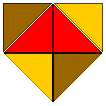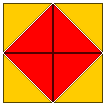[ Main page ]

# Mathematical commentary on YBC 7289

 There are a number of remarkable facts about the tablet, which is one of the very oldest mathematical diagrams extant. Given our vast ignorance about the era, speculation is inevitable. The Babylonians, unlike the early Greeks much later on, interpreted ratios of lengths as numbers. They possessed a place-value numbering system. It involved a principal base of 60, but the numbers from 0 to 59 were expressed in base 10. It is not apparent in the picture, but they didn't yet (i.e. in 1600 B.C.) possess a zero. Their representation system was a true floating point system: "30", for example, could be either 30 or 1/2. They weren't just finding a good (very good) approximation to the ratio of a diagonal to a side of a square. They knew that the ratio of the diagonal of a square to a side was a number whose square was 2. They possessed an algorithm for finding approximations to the square root of 2. It is the last point that has attracted most attention, but to one like myself, who has spent a great deal of time and effort thinking about graphical proofs of mathematical results, it is the second last point that is the most striking. I believe that we are looking here at the very origins of mathematical reasoning. This claim doesn't seem to be commonly accepted by mathematicians (see, for example, Jim Carlson's remarks in his notes on ancient mathematics), and historians might not appreciate it, but I don't see how else to interpret the figure itself. It amounts to a dissection of the square on the hypotenuse of an isosceles right triangle into pieces which can be reassembled to make up the two squares on the sides, and I can't see why the figure is exactly what it is if it weren't understood to demonstrate this. To make this idea slightly more plausible, consider the extrapolation of the figure on the tablet to the figure on the left below:Incidentally, the figure on the right, also closely related to our figure, is itself close to one of the figures found in the equally old tablet B.M. 15285. The second figure is also, remarkably enough, one of the diagrams that accompany Socrates' dialogue with the Greek boy in Plato's Meno, in which Socrates illustrates his claim that all knowledge is somehow innate to the human mind by drawing out of the boy a proof of Pythagoras' theorem for isosceles right triangles. So in effect, what we are seeing here is one of the very first examples of human reasoning - but visual reasoning, not verbal. Who are we to say how mathematical reasoning evolved? Or that for many purposes visual reasoning is not as valid as verbal?